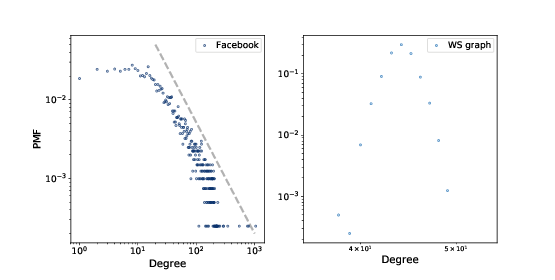# 6.5. Heavy-tailed Distributions¶Figure 6.2: PMF of degree in the Facebook dataset and in the WS model, on a log-log scale.¶

Heavy-tailed distributions are a common feature in many areas of complexity science and they will be a recurring theme of this book.

We can get a clearer picture of a heavy-tailed distribution by plotting it on a log-log axis, as shown in Figure 6.2. This transformation emphasizes the tail of the distribution; that is, the probabilities of large values.

Under this transformation, the data fall approximately on a straight line, which suggests that there is a power law relationship between the largest values in the distribution and their probabilities. Mathematically, a distribution obeys a power law if $$PMF(k) ∼ k−α$$ where PMF(k) is the fraction of nodes with degree k, α is a parameter, and the symbol ∼ indicates that the PMF is asymptotic to k−α as k increases.

If we take the log of both sides, we get:

$logPMF(k) ∼ −α logk$

So if a distribution follows a power law and we plot PMF(k) versus k on a log-log scale, we expect a straight line with slope −α, at least for large values of k.

All power law distributions are heavy-tailed, but there are other heavy-tailed distributions that don’t follow a power law. We will see more examples soon.

But first, we have a problem: the WS model has the high clustering and low path length we see in the data, but the degree distribution doesn’t resemble the data at all. This discrepancy is the motivation for our next topic, the Barabási-Albert model.